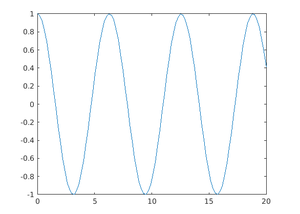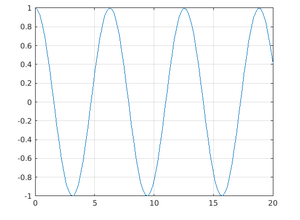Related Articles

# MATLAB Commands

• Last Updated : 04 Jul, 2021

MATLAB is an interactive multi-programming language and numeric computing environment developed by MathWorks. MATLAB provides the Commands that will be used when the user wants to interact with any application using the command line interface.

Following are the lists of commands used in MATLAB.

Example 1

## Matlab

 `% MATLAB Code to illustrate``% global``     ``function` `setGlobalx(val)``    ``global` `x`

Output:

`x = val;`

Example 2

## Matlab

 `% MATLAB Code to illustrate who``    ``x = 2``    ``who`

Output:

`Variables in the current scope: x`

Example 3

## Matlab

 `% MATLAB Code to illustrate``% clear``    ``clear``    ``type x`

Output:

`error: type 'x' is undefined`

Example 1

## Matlab

 `% MATLAB Code to illustrate``% pwd``    ``pwd`

Output:

`ans = /home/oo`

Example 2

## Matlab

 `% MATLAB Code to illustrate``% date``c = date`

Output:

`c= '18-jun-2021'`

Example 1

## Matlab

 `% MATLAB Code to illustrate``% disp`` ` `    ``A = [15 150];``    ``S = ``'Hello World.'``;``    ``disp(A)``    ``disp(S)`

Output:

```15 150

Hello World.```

Example 2

## Matlab

 `% MATLAB Code to illustrate``%input`` ``age = input(``'how old are you: '``); ``     ``% At this point, the variable: age, will contain ``     ``% whatever value the user types`

Output:

```20
```

Example 1

## Matlab

 `% MATLAB Code to illustrate ``%cat``    ``array1 = [1,2,3]``    ``array2 = [4,5,6]``    ``cat(1,array1,array2)`

Output:

```    ans =

1    2    3
4    5    6```

Example 2

## Matlab

 `% MATLAB Code to illustrate ``%max``max(array1)`

Output:

`ans = 3`

Example 3

## Matlab

 `%MATLAB Code to illustrate``%min``min(array2)`

Output:

`ans = 4`

## Matlab

 `%MATLAB Code to illustrate``%eye``eye(2,2)`

Output:

```    ans =
Diagonal Matrix
1    0
0    1```

Plotting Commands:

Example1:

## Matlab

 `% MATLAB Code to illustrate``%plot``    ``x = linspace(0,20)``    ``y = cos(x)``    ``plot(x,y)`

Output:Example 2:

## Matlab

 `%MATLAB Code to illustrate``%grid``    ``x = linspace(0,20)``    ``y = cos(x)``    ``plot(x,y)``    ``grid on`

Output:My Personal Notes arrow_drop_up